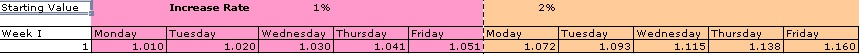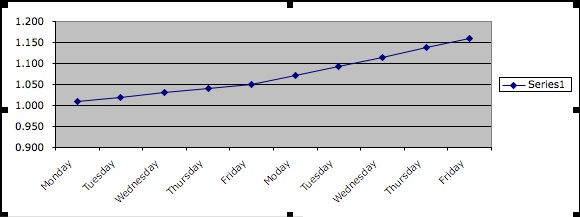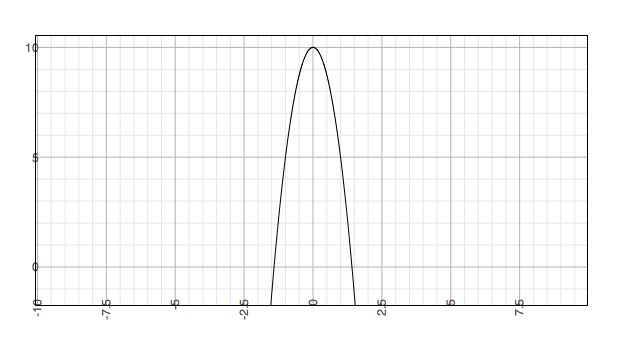Natural Science - Year I

#### Course Materials are always under revision! Weblecture content may change anytime prior to two weeks before scheduled chat session for content.Science Weblecture for Unit 21

 This Unit's Homework Page History Lecture Science Lecture Lab Parents' Notes

# Science Lecture for Unit 21: Medieval Mathematics

##### For Class
• Topic area: Concepts of rates and change
• Terms and concepts to know: periodicity, uniform motion, difform motion
• See historical period(s): Medieval Cosmology

## Computing

The late middle ages did not blindly accept the Aristotelian view of the universe, despite the attempts of ibn Rushd (Averroes) and Thomas Aquinas to reconcile their own experience and understanding of Christian doctrine with Aristotle's theory of moving objects, antiperistasis. One if the issues that they ran into was the problem of change and rates of change. Their work was a necessary step on the road to the development of Newton's calculus, a mathematical method for dealing with how one quantity changes as another related quantity changes.

#### Rates

Let's define rate as any change in anything with respect to time. The rise (or fall) of the stock market is a rate that sends delight or despair into investors. We can track the day-to-day changes of the Dow Jones industrial average, which is the price of a single stock averaged over thirty specific companies. Let's assume that the stock rises one percent each day for a week. At the end of the week, it will be some 5% plus a little more than it was at the beginning of the week. Then the next week, the stock goes up by 2% per day.In this case, the rate itself has changed, so we have two changes: the change in the average, and the change in the rate at which the average is changing. We can see this very easily if we plot the daily close values as a function of the day. The slope of the line indicates the rate of change. When the rate of change increases, the slope gets steeper.We've seen rate situations before when we talked about motion and acceleration in our earlier discussion of distance, velocity, and acceleration. You may recall that the distance you travel in a given period of time is a rate of change in displacement from some point in space. We sometimes write the change distancefinal - distanceinitial as df - di = Δd. The Greek letter Delta Δ is the mathematical symbol for difference or change in some value.

We can think of and symbolize a period of time the same way: we have a starting time tinitial and an ending time tfinal, and the difference is the period of time tfinal - tinitial = Δt that it takes us to go some distance Δd.

We can now express this as a rate: the change in position (equal to the distance traveled) during some period of time is Δd/Δt. Say that we walk four miles in an hour, then our Δd/Δt is 4 miles per hour, which we can write 4 mph. But you know what this is: it's speed or (if we throw in the direction we travel) velocity. So velocity is the rate of change in position with respect to time.

Now velocity itself can change. You've all heard advertisements for the performance of sports cars, when the manufacturer states that the car can go from 0 mph to 60 mph in 10 seconds. This is a change in what is already a rate (velocity); we call this change acceleration. It can be positive, so that velocity is increasing, or negative, in which case we may use the term deceleration to describe it.

Consider the situation of an object, like a ball, falling from a point 10 meters up, with just earth's gravitational acceleration to increase its speed. Earth's gravitational acceleration is a change in velocity over time, that is, ΔV/Δt. But ΔV is itself a change in distance over time, ΔD/Δt. Experiments show that a falling body increases its velocity by 9.8 meters/second each second that it falls. We express this acceleration as (9.8 meters/second)/(1 second) or 9.8 meters/second2. But this value is true only close to the surface of the earth. An object falling from far out in space experiences an increasing acceleration, so that acceleration itself is changing.The medieval critics of Aristotle recognized some difficulties with his discussion of motion, and they tried to distinguish unchanging velocity from changing velocity. They used the term uniform motion for constant velocity. This means the moving object continues moving, but does not speed up or slow down. Difform motion involves a velocity that is changing, so that the object is accelerating or decelerating. This change can itself be uniform (constant) or difform (changing). In reality, most situations involve motion where the speed is difformly difform: that is, velocity is changing but erratically. The medieval mathematicians realized that they could determine an average speed under these conditions, but they were frustrated in their attempts to determine the actual speed at a particular moment.

#### Limits

Aristotle's physics and mathematics challenged medieval astronomers in other ways. One of these was in the application of mathematical concepts to identify impossible situations and set limits on various quantities. This resulted in a specific debate over the size of the universe.Aristotle's argument was this. The Primum Mobile or outermost sphere rotates and provides motion for all the spheres below it. The sphere of the Primum Mobile had to make a complete rotation in some finite time, to generate motion through all the lower spheres to drive the spheres of the sun and moon to make their rotations in a day and a month, respectively.

Since the Primum Mobile was obviously larger than the sphere of the fixed stars, a point on the inner edge of the Primum Mobile would be traveling faster than Saturn. A point on the outer edge would be traveling even faster. If the cosmos were infinite in size, such a point would have to travel with infinite speed, a concept that to Aristotle made no sense, because it would destroy the concept of distance: such a point would be everywhere at once. Therefore the universe had to be finite in extent.

Another problem facing the medieval investigation of motion was what happens if we calculate a change in velocity at an instantaneous moment of time. If our definition of acceleration is ΔV/ΔT, and we make ΔT so small that it goes to zero, our division formula "blows up": x/0 = ∞, infinity. But we know that there is some real and finite velocity associated with each "moment" in time, so somehow, we need a mathematics that allows us to describe and calculate that value.

While the medieval philosophers could not find fault with Aristotle's reasons for claiming that the universe had to be finite, the conclusion still disturbed them, because it implied that God suffered limitations, and could not make a universe of any arbitrary size, including an infinite one. Their investigations of the application of limits raised many questions that could not be answered until Newton's invention of the calculus and the realization that the spheres of the heavens were not solid or connected.

### Study/Discussion Questions:

• Give one example each of uniformly uniform motion, uniformly difform motion, and difformly difform motion. Why is difformly uniform motion not possible?